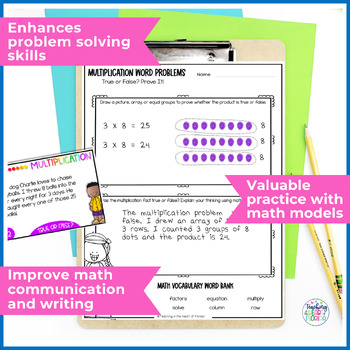# Multiplication Word Problems | Multiplication Task Cards | Error Analysis

Rated 4.81 out of 5, based on 188 reviews
188 Ratings;
3rd - 4th, Homeschool
Subjects
Resource Type
Standards
Formats Included
• PDF
Pages
14 pages
Report this resource to TPT
##### Also included in
1. These Multiplication and Division Word Problems Task Cards are a challenging and rigorous way for your 3rd and 4th-grade students to practice solving word problems through error analysis! Students will use math models to solve the word problem, then explain in writing what the error was and how they
Price \$7.60Original Price \$9.50Save \$1.90
2. If you're looking for a fun and engaging way to help your 3rd-grade students practice math skills in your elementary classroom, then these 3rd-grade Error Analysis Task Cards are perfect! Students will use error analysis to solve problems with addition, subtraction, multiplication, division, fractio
Price \$53.60Original Price \$67.00Save \$13.40

### Description

Make multiplication word problem practice fun and challenging for your 3rd and 4th grade students! These error analysis multiplication word problems will be an important part of math lessons and practice in math centers. Your elementary students will analyze multiplication word problems to find the errors, use math models to solve, and write to explain their solutions!

PLEASE SEE PREVIEW FOR A CLOSER LOOK!

───────────────────────────────

Included in these Multiplication Word Problems Task Card activities:

36 Multiplication Word Problems Task Cards: Extend your student’s understanding of multiplication with these task cards that are perfect for math centers or rotations.

Multiplication Math Error Analysis Worksheets: Students draw math models to find the error, then write to explain their thinking and prove their answers.

Multiplication Word Problems Challenge a Classmate Worksheet: Extend this multiplication practice with this fun collaborative activity for partners!

Scoot Recording Sheet: Use these task cards for a game of Scoot! This recording sheet keeps students organized as they play.

─────────────────────────────────

How can you use this Multiplication Word Problems Activities resource?

✔ Whole Group Instruction: Model this error analysis activity for your students. Teaching your students how to use error analysis will deepen their math understanding in a big way!

Small Group Instruction: These multiplication word problem task cards are very effective as a small group resource. You can model, then support students in this task. Adding the writing element to this task helps you see how your students are thinking and how they are solving problems.

Math Centers: This error analysis place value activity is a rigorous practice perfect for math centers or rotations.  Students are more engaged when math tasks are fun and challenging.

Formative Assessment or Exit Tickets: Want to know if your students have mastered multiplication? These task cards are an effective formative assessment. Use a quick exit ticket to get a snapshot of how your class comprehends multiplication word problems.

Partner Work: Students will be engaged and stay on task when working together on the Challenge a Classmate Activity! They love the challenge, plus it’s more fun when they work together!

Bellwork or Bellringers: Use these Multiplication Facts Practice task cards as a bellringer, and your students will become experts at multiplication!

────────────────────────────────

CLICK HERE to SAVE 20% on the Year-Long Error Analysis Bundle with all 3rd-grade math standards included!

This resource is also included in a Multiplication & Division Word Problems Error Analysis Mini-Bundle Pack!

────────────────────────────────

More resources for your MULTIPLICATION unit of study:

─────────────────────────────────

"Don't forget to leave feedback -you will receive TPT credits that you can use toward future purchases!

Thank you for visiting Teaching in the Heart of Florida!

Total Pages
14 pages
Included
Teaching Duration
Other
Report this resource to TPT
Reported resources will be reviewed by our team. Report this resource to let us know if this resource violates TPT’s content guidelines.

### Standards

to see state-specific standards (only available in the US).
Interpret products of whole numbers, e.g., interpret 5 × 7 as the total number of objects in 5 groups of 7 objects each. For example, describe a context in which a total number of objects can be expressed as 5 × 7.
Interpret whole-number quotients of whole numbers, e.g., interpret 56 ÷ 8 as the number of objects in each share when 56 objects are partitioned equally into 8 shares, or as a number of shares when 56 objects are partitioned into equal shares of 8 objects each. For example, describe a context in which a number of shares or a number of groups can be expressed as 56 ÷ 8.
Use multiplication and division within 100 to solve word problems in situations involving equal groups, arrays, and measurement quantities, e.g., by using drawings and equations with a symbol for the unknown number to represent the problem.
Solve two-step word problems using the four operations. Represent these problems using equations with a letter standing for the unknown quantity. Assess the reasonableness of answers using mental computation and estimation strategies including rounding.